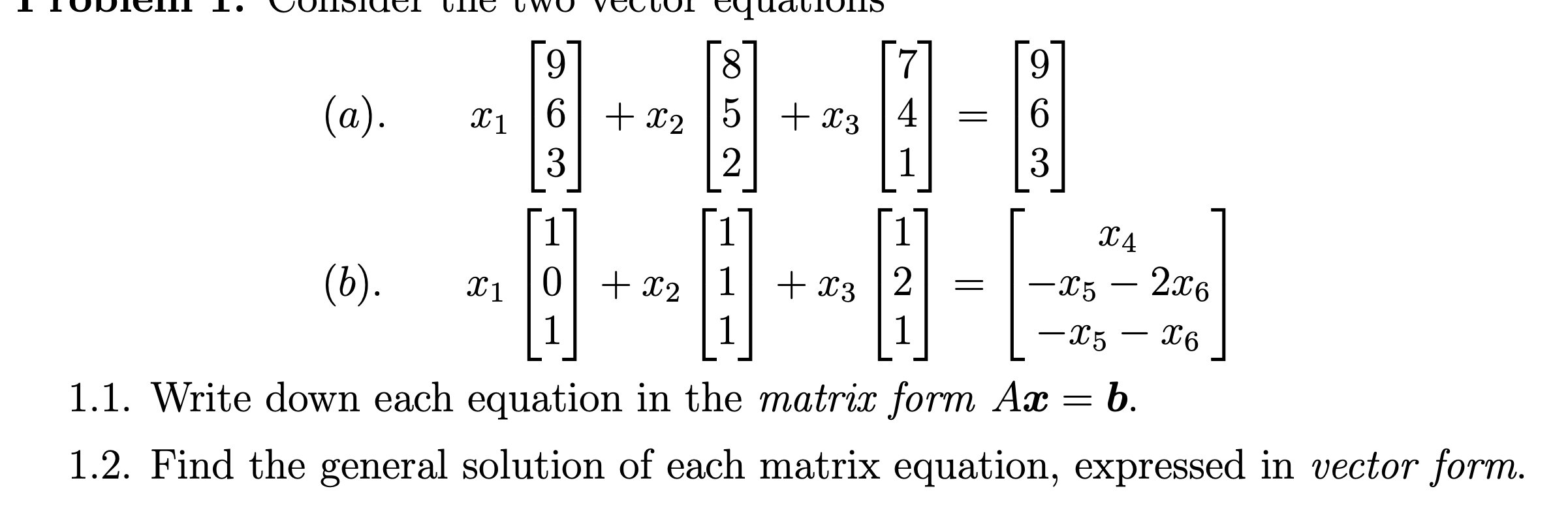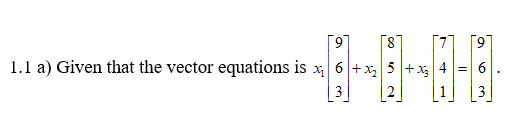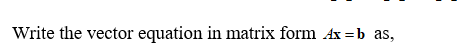# OIISIUETquatioIS8.6.(a).46.6| + x2 |5|+ x33X1%3D1X4– 2x60 + x2 |1|+ x3(b).-X5x111-X5X61.1. Write down each equation in the matrix form Ax = b.1.2. Find the general solution of each matrix equation, expressed in vector form.

Question
2 views

Screen shot questionhelp_outlineImage TranscriptioncloseOIISIUET quatioIS 8. 6. (a). 4 6. 6| + x2 |5|+ x3 3 X1 %3D 1 X4 – 2x6 0 + x2 |1|+ x3 (b). -X5 x1 1 1 -X5 X6 1.1. Write down each equation in the matrix form Ax = b. 1.2. Find the general solution of each matrix equation, expressed in vector form. fullscreen
check_circle

Step 1

Hey since there are multiple questions the first question is answered. Kindly repost if any particular question has to be answered.

Step 2Step 3...

### Want to see the full answer?

See Solution

#### Want to see this answer and more?

Solutions are written by subject experts who are available 24/7. Questions are typically answered within 1 hour.*

See Solution
*Response times may vary by subject and question.
Tagged in

### Math